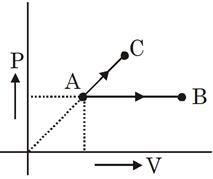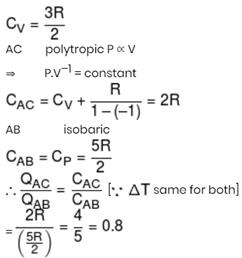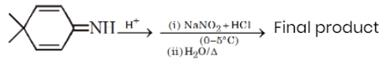Courses

# JEE Main Chemistry Mock - 7

## 25 Questions MCQ Test Mock Test Series for JEE Main & Advanced 2022 | JEE Main Chemistry Mock - 7

Description
This mock test of JEE Main Chemistry Mock - 7 for JEE helps you for every JEE entrance exam. This contains 25 Multiple Choice Questions for JEE JEE Main Chemistry Mock - 7 (mcq) to study with solutions a complete question bank. The solved questions answers in this JEE Main Chemistry Mock - 7 quiz give you a good mix of easy questions and tough questions. JEE students definitely take this JEE Main Chemistry Mock - 7 exercise for a better result in the exam. You can find other JEE Main Chemistry Mock - 7 extra questions, long questions & short questions for JEE on EduRev as well by searching above.
QUESTION: 1

Solution:
QUESTION: 2

### Which of the following is hypnotic ?

Solution:
Solution :
Hypnotic or soporific drugs, commonly known as sleeping pills, are a class of psychoactive drugs whose primary function is to induce sleep and to be used in the treatment of insomnia, or surgical anesthesia.
QUESTION: 3

### Enzymes are made up of

Solution:
QUESTION: 4
The order of decrease in atomic radii for Be, Na and Mg is
Solution: As we move from left to right in a period, nuclear charge increases while the number of the shells remains the same. Due to this, the valence electrons are pulled little closer to the nucleus and this results in a decrease of the atomic radius. For example, when we move from Li to Be, atomic size decreases. On the other hand, the atomic radii of elements increase with increase in atomic numbers as we move from top to bottom in a group.This is due to the addition of new energy shells in each succeeding element. Thus, as we move from Li to Na, atomic radius increases and when go from Na to Mg, atomic radius decreases but not as much as in case of Be.
QUESTION: 5

Which of the following gives blood red colour with KCNS ?

Solution:
[B]
Fe+3 give blood red cloour with KCNS.
[Fe(CNS)2]+
x − 2 =  + 1
x = + 3
QUESTION: 6

Given that
(i) C + O₂ → CO₂ ; ∆Ho = − xkJ,
(ii) 2CO + O₂ → 2CO₂ ; ∆Ho = −ykJ .
The enthalpy of formation of carbon monoxide will be

Solution:
QUESTION: 7
The rate at which a substance reacts depends on its
Solution:
QUESTION: 8
[Co(NH₃)₄(NO₂)₂]Cl exhibits
Solution:
QUESTION: 9
Strong electrolytes are those which
Solution:
QUESTION: 10
Which of the following reagent can be used to convert benzene diazonium chloride into benzene ?
Solution:
QUESTION: 11

In the electrochemical cell Pt, H(g) 1 atm ∕ H+(I M) ∕ Cu2+ (I M) ∕ Cu(s) . Which one of the following statements is true?

Solution:
QUESTION: 12

pH of the solution at 250C is 2. If the pH is to be doubled then the hydronium ion concentration of the solution should be

Solution:
QUESTION: 13
The pH of 10 M HCl aqueous solution is
Solution:
QUESTION: 14

Given that Ka for acetic acid as 1.8x10⁻5 and Kb of NH₄OH as 1.8x10⁻5 at 25oC,predict the nature of aqueous solution of ammonium acetate

Solution:
QUESTION: 15
Highest pH (14) is shown by
Solution:
QUESTION: 16
Which of the following compound does not react with metallic sodium?
Solution:  (B) is methyl ether, presumably water free, and unreactive under normal conditions. Of course, at room temperature, it will be a gas.
QUESTION: 17
Which of the following polymer is formed when caprolactum is heated with the traces of water?
Solution:
QUESTION: 18
Ethane is obtained from ethylene by which of the following methods?
Solution:
QUESTION: 19
Which of the following is an example of body centred cube?
Solution:
QUESTION: 20
Which of the following metal oxides is antiferromagnetic in nature?
Solution:
*Answer can only contain numeric values
QUESTION: 21

One mole ideal monoatomic gas is heated in two different processes according to path AB and AC. If temperature of state B and state C are equal. Calculate qAC/qABSolution:*Answer can only contain numeric values
QUESTION: 22

What is the molecular weight of the final product in the following reaction sequenceSolution:
*Answer can only contain numeric values
QUESTION: 23

6.84 gm Al2(SO4)3 is needed to coagulate 2.5 L of As2S3 sol completely in 2.0 hrs. The coagulation value of Al2(SO4)3 in terms of millimoles per litre is :
[Atomic mass : Al = 27, S = 32].

Solution:
*Answer can only contain numeric values
QUESTION: 24

An ionic compound (A+B) crystallizes in rock salt structure. If the ionic radii of A+ and B is 200 pm and 400 pm respectively, then calculate distance between nearest anions in Å.

Solution:
*Answer can only contain numeric values
QUESTION: 25

The sum of Number of unpaired electrons in
[COCl6]–3, [Cr(NH3)6]+3 , [Zn(NH3)4]+2 →

Solution:

Track your progress, build streaks, highlight & save important lessons and more!

### Similar Content### Related tests